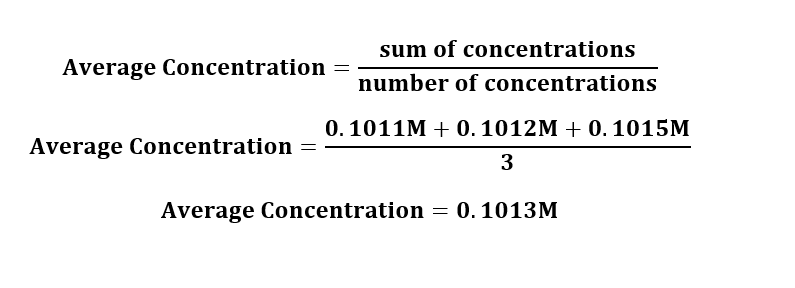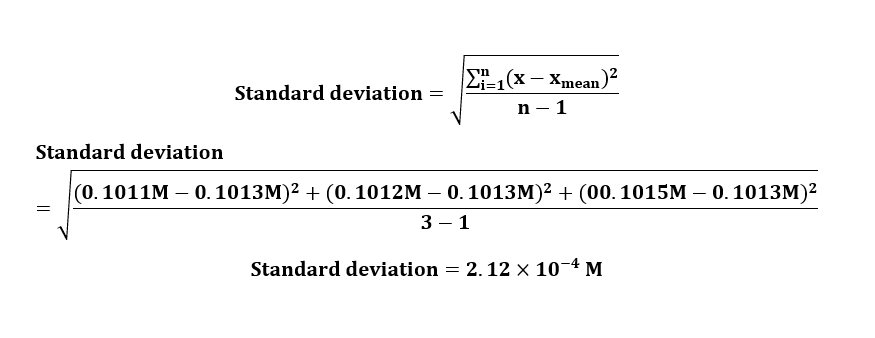# Can you show me how to calculate this?You have calculated the [NaOH] for three trials shown below;.1011M, .1012M, .1015MCalculate the %RSD. A 0.3% RSD or less is required. Based on your calculations should 1 more trial be performed?

Question
1 views

Can you show me how to calculate this?

You have calculated the [NaOH] for three trials shown below;

.1011M, .1012M, .1015M

Calculate the %RSD. A 0.3% RSD or less is required. Based on your calculations should 1 more trial be performed?

check_circle

Step 1

Given:

Concentrations: .1011M, .1012M, .1015M

Step 2

Calculation Average (mean) of concentrations:Step 3

Calculation Standard deviation of concentrations:...

### Want to see the full answer?

See Solution

#### Want to see this answer and more?

Solutions are written by subject experts who are available 24/7. Questions are typically answered within 1 hour.*

See Solution
*Response times may vary by subject and question.
Tagged in

### Chemistry